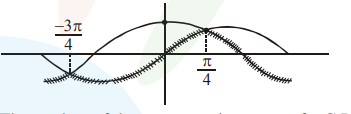# Let S be the set of all points in`
Question:

Let $S$ be the set of all points in $(-\pi, \pi)$ at which the function, $\mathrm{f}(\mathrm{x})=\min \{\sin x, \cos \mathrm{x}\}$ is not differentiable. Then $S$ is a subset of which of the following?

1. $\left\{-\frac{3 \pi}{4},-\frac{\pi}{4}, \frac{3 \pi}{4}, \frac{\pi}{4}\right\}$

2. $\left\{-\frac{3 \pi}{4},-\frac{\pi}{2}, \frac{\pi}{2}, \frac{3 \pi}{4}\right\}$

3. $\left\{-\frac{\pi}{2},-\frac{\pi}{4}, \frac{\pi}{4}, \frac{\pi}{2}\right\}$

4. $\left\{-\frac{\pi}{4}, 0, \frac{\pi}{4}\right\}$

Correct Option: 1

Solution: